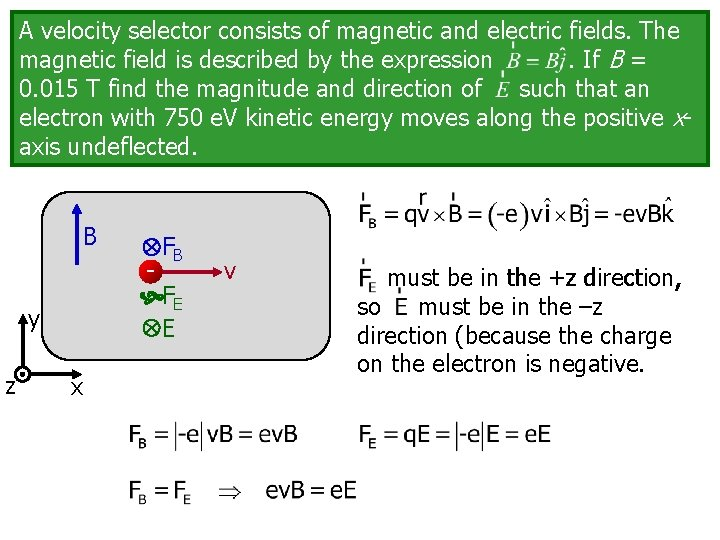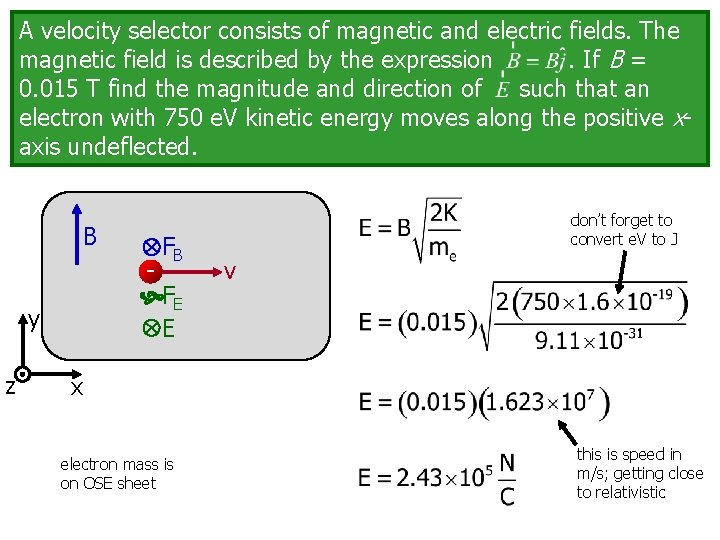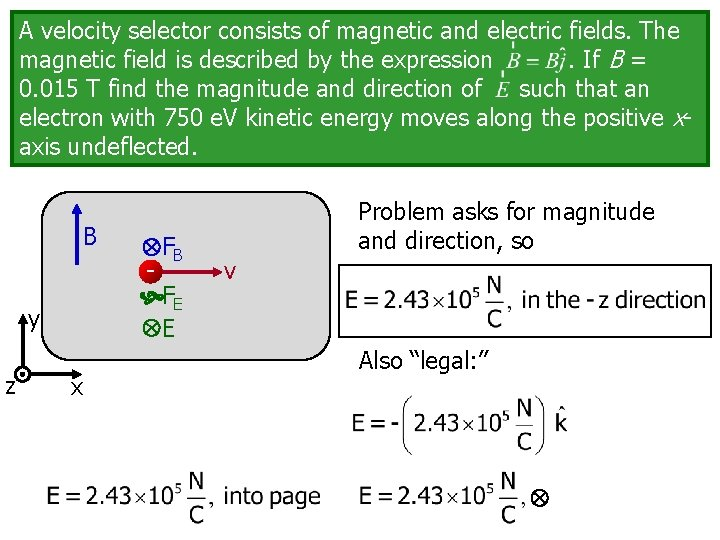# A velocity selector consists of magnetic and electric

• Slides: 4A velocity selector consists of magnetic and electric fields. The magnetic field is described by the expression. If B = 0. 015 T find the magnitude and direction of such that an electron with 750 e. V kinetic energy moves along the positive xaxis undeflected. B y z x FB FE E v must be in the +z direction, so must be in the –z direction (because the charge on the electron is negative.A velocity selector consists of magnetic and electric fields. The magnetic field is described by the expression. If B = 0. 015 T find the magnitude and direction of such that an electron with 750 e. V kinetic energy moves along the positive xaxis undeflected. B y z x FB FE E vA velocity selector consists of magnetic and electric fields. The magnetic field is described by the expression. If B = 0. 015 T find the magnitude and direction of such that an electron with 750 e. V kinetic energy moves along the positive xaxis undeflected. B y z FB FE E don’t forget to convert e. V to J v x electron mass is on OSE sheet this is speed in m/s; getting close to relativisticA velocity selector consists of magnetic and electric fields. The magnetic field is described by the expression. If B = 0. 015 T find the magnitude and direction of such that an electron with 750 e. V kinetic energy moves along the positive xaxis undeflected. B y z x FB FE E Problem asks for magnitude and direction, so v Also “legal: ”